# RBSE Maths Class 9 Chapter 4: Important Questions and Solutions

RBSE Maths Chapter 4 – Linear Equations in Two Variables Class 9 Important questions and solutions are available here. The important questions and solutions of chapter 4 available at BYJU’S have detailed explanations, along with required graphs. These RBSE Class 9 solutions will help the students to improve their problem solving and analytical skills.

Chapter 4 of the RBSE Class 9 Maths will help the students to solve the given pair of linear equations using different methods. After practicing these important questions, students will be able to understand the graphical method of solving, various algebraic methods of solving the given system of equations. Besides, they will also learn how to form linear equations for word problems.

### RBSE Maths Chapter 4: Exercise 4.1 Textbook Important Questions and Solutions

Question 1: Solve the following equations graphically:

x + 3y = 6

2x – 3y = 12

Solution:

Given equations are:

x + 3y = 6

2x – 3y = 12

Consider the first equation,

x + 3y = 6

3y = -x + 6

y = (-⅓)x + 2 ….(i)

Table for equation (i),

 x 0 6 y 2 0

Now consider another equation,

2x – 3y = 12

3y = 2x – 12

y = (⅔)x – 4 ….(ii)

Table for equation (ii)

 x 0 6 y -4 0

Graph: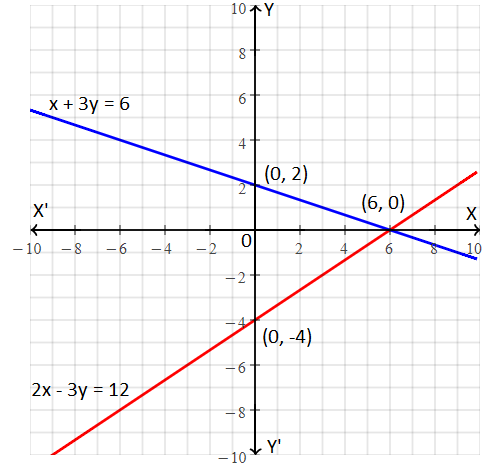By plotting the corresponding points on the coordinate plane as shown in the above figure, the point of intersection of two lines obtained as (6, 0)

Hence, (6, 0) is the solution for a given pair of linear equations.

Question 2: Solve the following equations graphically:

x – 2y = 6

3x – 6y = 0

Solution:

Given linear equations are:

x – 2y = 6

3x – 6y = 0

Consider the first equation,

x – 2y = 6

2y = x – 6

y = (½)x – 6 ….(i)

Table for equation (i)

 x 0 2 6 y 3 -2 0

Now, consider another equation,

3x – 6y = 0

6y = 3x

Y = (½)x ….(ii)

Table for equation (ii)

 x 0 4 -4 y 0 2 -2

Graph: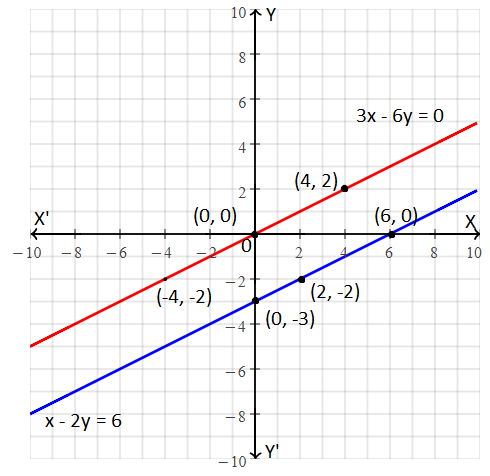By plotting the corresponding points on the coordinate plane as shown in the above figure, there is no point of intersection since the two lines are parallel to each other.

Hence, there is no solution for a given pair of linear equations.

Question 3: Solve the following equations graphically:

2x – 3y + 13 = 0

3x – 2y + 12 = 0

Solution:

Given linear equations are:

2x – 3y + 13 = 0

3x – 2y + 12 = 0

Considering the first equation,

2x – 3y + 13 = 0

3y = 2x + 13

y = (⅔)x + (13/3) ….(i)

Table for equation (i):

 x -8 -2 4 y -1 3 7

Now, considering another equation,

3x – 2y + 12 = 0

2y = 3x + 12

y = (3/2)x + 6 ….(ii)

Table for equation (ii):

 x -4 -2 0 y 0 3 6

Graph: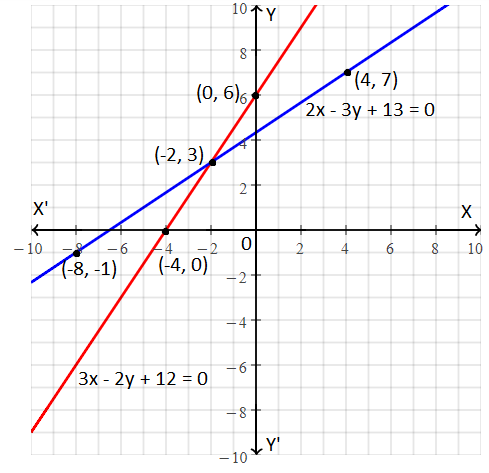By plotting the corresponding points on the coordinate plane as shown in the above figure, the point of intersection of two lines obtained as (-2, 3)

Hence, (-2, 3) is the solution for a given pair of linear equations.

Question 4: Solve the following equations graphically:

3x – 4y = 1

-2x + (8/3)y = 5

Solution:

Given linear equations are:

3x – 4y = 1

-2x + (8/3)y = 5

Considering the first equation,

3x – 4y = 1

4y = 3x – 1

y = (¾)x – ¼ ….(i)

Table for equation (i):

 x -1 3 7 y -1 2 5

Now, considering another equation,

-2x + (8/3)y = 5

(8/3)y = 2x + 5

8y = 6x + 15

y = (6/8)x + (15/8)

y = (¾)x + (15/8) ….(ii)

Table for equation (ii):

 x -4 0 8 y 1.1 1.8 7.9

Graph: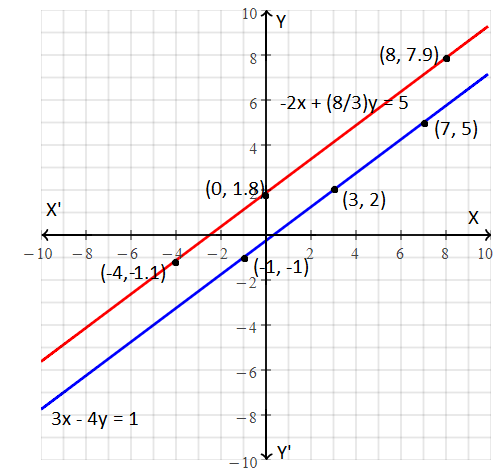By plotting the corresponding points on the coordinate plane as shown in the above figure, there is no point of intersection since the two lines are parallel to each other.

Hence, there is no solution for a given pair of linear equations.

Question 5: Solve the following equations graphically:

3x – y = 2

6x – 2y = 4

Solution:

Given linear equations are:

3x – y = 2

6x – 2y = 4

Considering the first equation,

3x – y = 2

y = 3x – 2 ….(i)

Now, considering another equation,

6x – 2y = 4

2(3x – y) = 4

3x – y = 4/2

3x – y = 2

y = 3x – 2 ….(ii)

Thus, equation (i) and (ii) are the same.

Table for the given equations:

 x 0 1 2 y 2 1 4

Graph: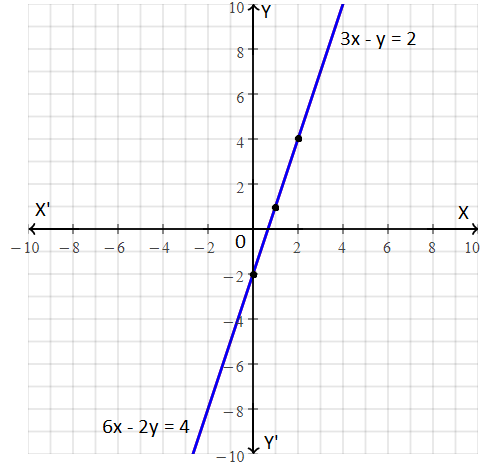By plotting the corresponding points on the coordinate plane as shown in the above figure, we can observe that the two lines coincide.

Hence, there exist infinitely many solutions for a given pair of linear equations.

### RBSE Maths Chapter 4: Exercise 4.2 Textbook Important Questions and Solutions

Question 6: Solve the following equations by the method of elimination (by substitution):

x + 2y = -1

2x – 3y = 12

Solution:

Given linear equations are:

x + 2y = -1 ….(i)

2x – 3y = 12 ….(ii)

From equation (i),

x + 2y = -1

x = -1 – 2y ….(iii)

Substituting equation (iii) in (ii),

2(-1 – 2y) – 3y = 12

-2 – 4y – 3y = 12

-7y = 12 + 2

-7y = 14

y = 14/(-7)

y = -2

Now substituting y = -2 in (iii),

x = -1 – 2(-2)

x = -1 + 4

x = 3

Therefore, the solution for a given pair of linear equations is x = 3 and y = -2.

Question 7: Solve the following equations by the method of elimination (by substitution):

3x + 2y = 11

2x + 3y = 4

Solution:

Given linear equations are:

3x + 2y = 11 ….(i)

2x + 3y = 4 ….(ii)

From equation (ii),

2x + 3y = 4

2x = 4 – 3y

x = (4/2) – (3/2)y

x = 2 – (3/2)y ….(iii)

Substituting equation (iii) in (i),

3[2 – (3/2)y] + 2y = 11

6 – (9/2)y + 2y = 11

-(9/2)y + 2y = 11 – 6

[(-9 + 4)/ 2]y = 5

(-5/2)y = 5

y = (-⅖) × 5

y = -2

Substituting y = -2 in equation (iii),

x = 2 – (3/2) × (-2)

x = 2 + 3

x = 5

Therefore, the solution for a given pair of linear equations is x = 5 and y = -2.

Question 8: Solve the following equations by the method of elimination by equating the coefficients:

0.4x + 0.3y = 1.7

0.7x – 0.2y = 0.8

Solution:

Given linear equations are:

0.4x + 0.3y = 1.7 ….(i)

0.7x – 0.2y = 0.8 ….(ii)

Multiplying equation (i) with 2,

0.8x + 0.6y = 3.4 ….(iii)

Multiplying equation (ii) with 3,

2.1x – 0.6y = 2.4 ….(iv)

0.8x + 0.6y + 2.1x – 0.6y = 3.4 + 2.4

2.9x = 5.8

x = (5.8)/(2.9)

x = 2

Substituting x = 2 in equation (i),

(0.4)2 + 0.3y = 1.7

0.8 + 0.3y = 1.7

0.3y = 1.7 – 0.8

0.3y = 0.9

y = (0.9)/(0.3)

y = 3

Therefore, the solution for a given pair of linear equations is x = 2 and y = 3.

Question 9: Solve the following equations by the method of elimination by equating the coefficients:

2x + y = 13

5x – 3y = 16

Solution:

Given,

2x + y = 13 ….(i)

5x – 3y = 16 ….(ii)

Multiplying equation (i) with 3,

6x + 3y = 39 ….(iii)

5x – 3y + 6x + 3y = 16 + 39

11x = 55

x = 55/11

x = 5

Substituting x = 5 in equation (i),

2(5) + y = 13

10 + y = 13

y = 13 – 10

y = 3

Therefore, the solution for a given pair of linear equations is x = 5 and y = 3.

Question 10: Solve the following equations by the method of elimination by equating the coefficients:

x + 2y = 3/2

2x + y = 3/2

Solution:

Given,

x + 2y = 3/2 ….(i)

2x + y = 3/2 ….(ii)

Multiplying equation (i) with 2,

2x + 4y = (3/2) × 2

2x + 4y = 3 ….(iii)

Subtracting (ii) from (iii),

2x + 4y -(2x + y) = 3 – (3/2)

4y – y = (6 – 3)/2

3y = 3/2

y = (3/2) × (⅓)

y = ½

Substituting y = ½ in equation (i),

x + 2(½) = 3/2

x + 1 = 3/2

x = (3/2) – 1

x = (3 – 2)/2

x = ½

Therefore, the solution for a given pair of linear equations is x = 1/2 and y = 1/2.

Question 11: Solve the following equations:

8v – 3u = 5uv

6v – 5u = -2uv

Solution:

Given equations are:

8v – 3u = 5uv ….(i)

6v – 5u = -2uv ….(ii)

Multiplying equation (i) with 5,

40v – 15u = 25uv ….(iii)

Multiplying equation (ii) with 3,

18v – 15u = -6uv

Subtracting (iv) from (iii),

(40v – 15v) – (18v – 15u) = 25uv – (-6uv)

22v = 31uv

u = (22v/31v)

u = 22/31

Substituting u = 23/31 in equation (i)

8v – 3(22/31) = 5v(22/31)

8v – (66/31) = 110v/31

8v – (110/31)v = 66/31

(248 – 110)v/31 = 66/31

138v = 66

v = 66/138

v = 11/23

Therefore, the solution for a given pair of linear equations is u = 22/31 and v = 11/23.

Question 12: Solve the following equations:

$$\begin{array}{l}\frac{5}{x+y}-\frac{2}{x-y}=-1\\ \frac{15}{x+y}+\frac{7}{x-y}=10\end{array}$$

Solution:

Given,

$$\begin{array}{l}\frac{5}{x+y}-\frac{2}{x-y}=-1….(i)\\ \frac{15}{x+y}+\frac{7}{x-y}=10….(ii)\end{array}$$

Let us assume that, 1/(x + y) = a and 1/(x – y) = b

Substituting a and b in equation (i) and (ii),

5a – 2b = -1 ….(iii)

15a + 7b = 10 ….(iv)

Multiplying equation (iii) with 3,

15a – 6b = -3 ….(v)

Subtracting (iv) from (v),

15a – 6b – (15a + 7b) = -3 – 10

-6b – 7b = -13

-13b = -13

b = 13/13

b = 1

Substituting b = 1 in equation (iii),

5a – 2(1) = -1

5a = -1 + 2

5a = 1

a = ⅕

We know that,

1/(x + y) = a = ⅕

Thus, x + y = 5 ….(vi)

1/(x – y) = b = 1

x – y = 1 ….(vii)

2x = 5 + 1

2x = 6

x = 6/2 = 3

Substituting x = 3 in equation (vi),

3 + y = 5

y = 5 – 3 = 2

Therefore, the solution for a given pair of linear equations is x = 3 and y = 2.

### RBSE Maths Chapter 4: Exercise 4.3 Textbook Important Questions and Solutions

Question 13: Solve the following equation by cross-multiplication method.

8x + 5y = 9

3x + 2y = 4

Solution:

Given equations are:

8x + 5y = 9

3x + 2y = 4

These equations can be written as:

8x + 5y – 9 = 0

3x + 2y – 4 = 0

Comparing with the standard form,

a1 = 8, b1 = 5, c1 = -9

a2 = 3, b2 = 2, c2 = -4

Using cross-multiplication method,

x/(b1c2 – b2c1) = y/(c1a2 – c2a1) = 1/(a1b2 – a2b1)

x/[5(-4) – 2(-9)] = y/[(-9)3 – (-4)8] = 1/[8(2) – 3(5)]

x/(-20 + 18) = y/(-27 + 32) = 1/(16 – 15)

x/(-2) = y/5 = 1

Thus, x = -2, y = 5

Question 14: Check the equations given below, if they have a unique solution, no solution or infinitely many solutions. In case there is a unique solution, find it.

2x – y = 6

x – y = 2

Solution:

Given equations are:

2x – y = 6 ….(i)

x – y = 2 ….(ii)

Comparing with the standard form,

a1 = 2, b1 = -1, c1 = -6

a2 = 1, b2 = -1, c2 = -2

a1/a2 = 2/1 = 2

b1/b2 = -1/-1 = 1

a1/a2 ≠ b1/b2

Hence, the given linear equations have a unique solution.

From equation (ii),

x = y + 2 ….(iii)

Substituting (iii) in (i),

2(y + 2) – y = 6

2y + 4 – y = 6

y = 6 – 4 = 2

Substituting y = 2 in (iii),

x = 2 + 2 = 4

Therefore, the required solution is x = 4 and y = 2.

Question 15: Check the equations given below, if they have a unique solution, no solution or infinitely many solutions. In case there is a unique solution, find it.

x + 2y + 1 = 0

2x – 3y – 12 = 0

Solution:

Given,

x + 2y + 1 = 0 ….(i)

2x – 3y – 12 = 0 ….(ii)

Comparing with the standard form,

a1 = 1, b1 = 2, c1 = 1

a2 = 2, b2 = -3, c2 = -12

a1/a2 = 1/2

b1/b2 = 2/(-3) = -2/3

a1/a2 ≠ b1/b2

Hence, the given linear equations have a unique solution.

From equation (i),

x = -1 – 2y ….(iii)

Substituting equation (iii) in (ii),

2(-1 – 2y) – 3y – 12 = 0

-2 – 4y – 3y – 12 = 0

-7y – 14 = 0

7y = -14

y = -14/7 = -2

Substituting y = -2 in (iii),

x = -1 – 2(-2)

x = -1 + 4 = 3

Therefore, the required solution is x = 3 and y = -2.

Question 16: If the following system of equations has no solution, find the value of k.

2x + ky = 1

3x – 5y = 7

Solution:

Given,

2x + ky = 1

3x – 5y = 7

Comparing with the standard form,

a1 = 2, b1 = k, c1 = -1

a2 = 3, b2 = -5, c2 = -7

Condition for no solution:

a1/a2 = b1/b2 ≠ c1/c2

⅔ = -k/5 ≠ ⅕

-k/5 = ⅔

k = -10/3

Question 17: Find the solution of system of equations: mx – ny = m2 + n2; x + y = 2m

Solution:

Given system of equations:

mx – ny = m2 + n2 ….(i)

x + y = 2m ….(ii)

Multiplying equation (ii) with n,

nx + ny = 2mn ….(iii)

mx – ny + nx + ny = m2 + n2 + 2mn

(m + n)x = (m + n)2

x = (m + n)2/(m + n) = m + n

Substituting x = m + n in equation (ii),

m + n + y = 2m

y = 2m – m – n

y = m – n

Hence, the required solution is x = m + n and y = m – n.

### RBSE Maths Chapter 4: Exercise 4.4 Textbook Important Questions and Solutions

Question 18: In a two-digit number, the one’s digit is 3 times the ten’s digit. If 10 is added to the two times of the number, its digits interchange their places in the new number. Find the number.

Solution:

Let the two digits number be 10x + y ….(i)

According to the given,

y = 3x ….(ii)

And

2(10x + y) + 10 = 10y + x

20x + 2y + 10 = 10y + x

20x + 2y – 10y – x = -10

19x – 8y = -10 ….(iii)

Substituting equation (ii) in (iii),

19x – 8(3x) = -10

19x – 24x = -10

-5x = -10

x = 10/5 = 2

Substituting x = 2 in (ii),

y = 3(2) = 6

Substituting the values of x and y in (i),

10(2) + 6 = 20 + 6 = 26

Therefore, the number is 26.

Question 19: The ratio of two numbers is 3 : 4. If 5 is subtracted from each of the numbers, then the ratio becomes 5 : 7. Find the numbers.

Solution:

Let the two numbers be x and y, respectively.

According to the given,

x : y = 3 : 4

Thus, x = 3k, y = 4k, where k is proportionality constant.

Also,

(x – y) : (y – 5) = 5 : 7

(x – 5)/(y – 5) = 5/7

7(x – 5) = 5(y – 5)

7x – 35 = 5y – 25

Substituting x = 3k and y = 4k in the above equation,

7(3k) – 35 = 5(4k) – 25

21k – 20k = 35 – 25

k = 10

Therefore, x = 3k = 3 × 10 = 30

y = 4 × 10 = 40

Therefore, the required numbers are 30 and 40, respectively.

Question 20: The cost of 4 chairs and 3 tables is Rs. 2100 and the cost of 5 chairs and 2 tables is Rs. 1750. Find the cost of one chair and one table separately.

Solution:

Let the cost of a chair be Rs. x and the cost of a table be Rs. y, respectively.

According to the given,

4x + 3y = 2100 ….(i)

5x + 2y = 1750 ….(ii)

Multiplying equation (i) with 2,

8x + 6y = 4200 ….(iii)

Multiplying equation (ii) with 3,

15x + 6y = 5250 ….(iv)

Subtracting (iii) from (iv),

7x = 1050

x = 1050/7 = 150

Substituting x = 150 in (i),

4(150) + 3y = 2100

3y = 2100 – 600

3y = 1500

y = 1500/3 = 500

Therefore, the cost of one chair is Rs. 150 and the cost of one table is Rs. 500.

Question 21: If 3 times of a larger number is divided by a smaller number the quotient is 4 and the remainder is 3 and when 7 times of the smaller number is divided by the larger number, then the quotient is 5 and the remainder is 1. Find the two numbers.

Solution:

Let x be the larger number and y be the smaller number.

According to the given,

3x = 4y + 3

3x – 4y = 3 ….(i)

And

7y = 5x + 1

-5x + 7y = 1 ….(ii)

Multiplying equation (i) with 5,

15x – 20y = 15 ….(iii)

Multiplying equation (ii) with 3,

-15x + 21y = 3 ….(iv)

y = 18

Substituting y = 18 in (i),

3x – 4(18) = 3

3x = 72 + 3

3x = 75

x = 75/3 = 25

Therefore, 25 is the larger number and 18 is the smaller number.

Question 22: 5 years ago Geeta’s age was 3 times of Kamla’s age. After 10 year Geeta’s age will be 2 times of Kamla’s age. Find their present ages.

Solution:

Let the present age of Geeta be x years and Kamla’s be y years.

5 years ago, their ages were (x – 5) and (y – 5), respectively.

According to the given,

(x – 5) = 3(y – 5)

x – 5 = 3y – 15

x – 3y = 5 – 15

x – 3y = -10 ….(i)

10 years hence, their ages will be (x + 10) and (y + 10) years respectively.

Now,

x + 10 = 2(y + 10)

x + 10 = 2y + 20

x – 2y = 20 – 10

x – 2y = 10 ….(ii)

Subtracting (i) from (ii),

-2y – (-3y) = 10 – (-10)

y = 20

Substituting y = 20 in equation (ii)

x – 2(20) = 10

x – 40 = 10

x = 40 + 10 = 50

Therefore, the present ages of Geeta and Kamla are 50 years and 20 years, respectively.

### RBSE Maths Chapter 4: Additional Important Questions and Solutions

Question 1: Find the solution of the following equations using the graphical method.

x + y = 3

3x + 2y = 7

Solution:

Given,

x + y = 3

3x + 2y = 7

Considering the first equation,

x + y = 3

y = 3 – x ….(i)

Table for equation (i)

 x 0 1 3 y 3 2 0

Now, considering another equation,

3x + 2y = 7

2y = 7 – 3x

y = 7/2 – (3/2)x ….(ii)

Table for equation (ii)

 x 0 1 3 y 3.5 2 -1

Graph: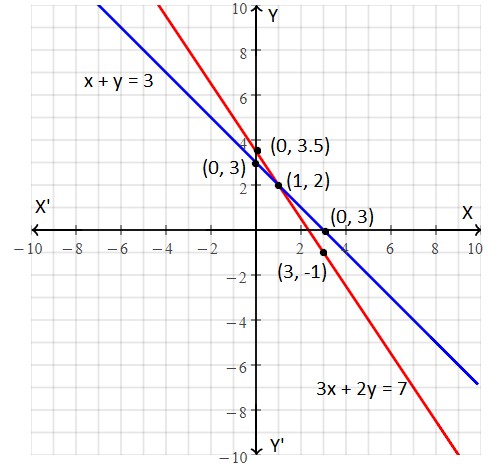By plotting the corresponding points on the coordinate plane as shown in the above figure, the point of intersection of two lines obtained as (1, 2)

Hence, (1, 2) is the solution for a given pair of linear equations.

Question 2: Express the following linear equations in the form ax + by + c = 0 and indicate the value of a, b and c.

(i) 4 = 5x – 3y (ii) x = 3y

Solution:

(i) 4 = 5x – 3y

5x – 3y – 4 = 0

This is of the form ax + by + c = 0.

Therefore, a = 5, b = -3 and c = -4.

(ii) x = 3y

x – 3y = 0

Or

x – 3y + 0 = 0

This is of form ax + by + c = 0.

Therefore, a = 1, b = -3 and c = 0

Question 3: Draw the graph of the linear equation x + y = 7

Solution:

Given equation is:

x + y = 7

y = 7 – x

Table for the corresponding values of x and y:

 x 0 7 y 7 0

Graph: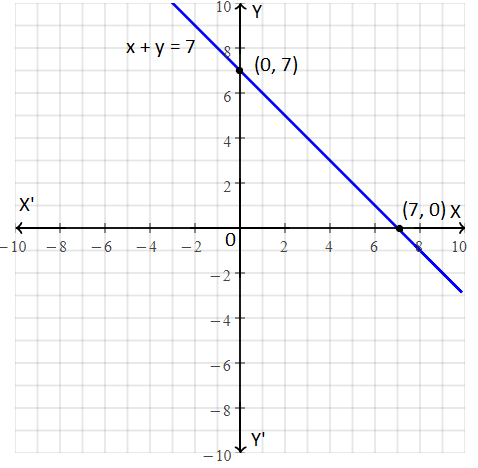Question 4: If 3x – 2y + 7 = 0 and x = 2, then find the value of y.

Solution:

Given,

3x – 2y + 7 = 0….(i)

x = 2 ….(ii)

Substituting (ii) in (i),

3(2) – 2y + 7 = 0

6 – 2y + 7 = 0

13 – 2y = 0

2y = 13

y = 13/2

Or

y = 6.5

Question 5: Solve the following equations by graphical method:

x + 2y = 5

2x + y = 4

Solution:

Given,

x + 2y = 5

2x + y = 4

Considering the first equation,

x + 2y = 5

2y = 5 – x

y = (5/2) – (½)x ….(i)

Table for equation (i),

 x 0 1 5 y 2.5 2 0

Now, considering the another equation,

2x + y = 4

y = 4 – 2x ….(ii)

Table for equation (ii),

 x 0 1 2 y 4 2 0

Graph: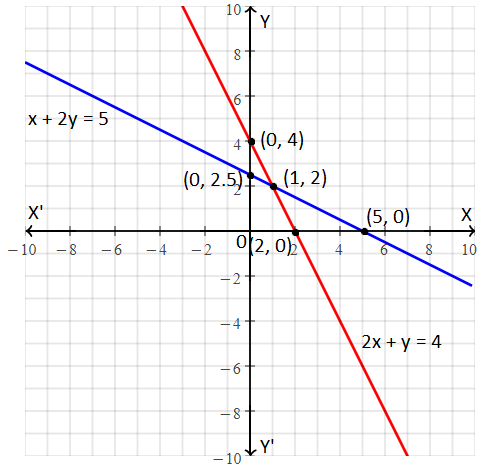By plotting the corresponding points on the coordinate plane as shown in the above figure, the point of intersection of two lines obtained as (1, 2)

Hence, (1, 2) is the solution for a given pair of linear equations.

Question 6: The perimeter of a rectangle is 56 cm. The ratio of length and its breadth is 4 : 3. Find the length and breadth of the rectangle.

Solution:

Let the length and breadth of the rectangle be x and y units, respectively.

According to the given,

Perimeter of rectangle:

2(x + y) = 56

x + y = 28 ….(i)

Given, the ratio of length and breadth of the rectangle is 4 : 3.

Let x = 4k, y = 3k

Substituting x = 4k and y = 3k in equation (i),

4k + 3k = 28

7k = 28

k = 28/7 = 4

Thus, x = 4(4) = 16

y = 3(4) = 12

Therefore, the length and breadth of the rectangle are 16 units and 12 units, respectively.

Question 7: Find the value of λ if the system of equations 3x + λy + 1 = 0, 2x + y – 9 = 0

(i) has a unique solution

(ii) has no solution.

Solution:

Given system of equations:

3x + λy + 1 = 0

2x + y – 9 = 0

Comparing with the standard form,

a1 = 3, b1 = λ, c1 = 1

a2 = 2, b2 = 1, c2 = -9

(i) Condition for unique solution is:

a1/a2 ≠ b1/b2

3/2 ≠ λ/1

λ ≠ 3/2

Hence, λ takes all the real numbers except 3/2 to have a unique solution.

(ii) Condition for no solution is:

a1/a2 = b1/b2 ≠ c1/c2

3/2 = λ/1 ≠ -1/9

λ = 3/2

Therefore, for λ = 3/2, the given system of equations has no solution.

Question 8: What is the condition of the system of linear equations in two variables to have infinitely many solutions?

Solution:

Let a1x + b1y + c1 = 0 and a2x + b2y + c2 = 0 be the pair of linear equations in two variables. And the condition for having infinitely many solutions is a1/a2 = b1/b2 = c1/c,2.

Question 9: Solve the following equations by the method of elimination by equating the coefficients:

$$\begin{array}{l}\frac{x}{7}+\frac{y}{3}=5\\ \frac{x}{2}-\frac{y}{9}=6\end{array}$$

Solution:

Given,

$$\begin{array}{l}\frac{x}{7}+\frac{y}{3}=5….(i)\\ \frac{x}{2}-\frac{y}{9}=6….(ii)\end{array}$$

Multiplying equation (i) with ⅓,

$$\begin{array}{l}\frac{x}{21}+\frac{y}{9}=\frac{5}{3}….(iii)\end{array}$$

$$\begin{array}{l}\frac{x}{21}+\frac{y}{9}+\frac{x}{2}-\frac{y}{9}=\frac{5}{3}+6\end{array}$$

(x/21) + (x/2) = (5 + 18)/3

(2x + 21x)/42 = 23/3

23x/42 = 23/3

x = 42/3 = 14

Substituting x = 14 in equation (ii),

(14/2) – (y/9) = 6

7 – (y/9) = 6

y/9 = 7 – 6

y/9 = 1

y = 9

Therefore, the solution for the given system of equations is x = 14 and y = 9.

Question 10: Solve the following equations by the method of cross-multiplication:

2x + 3y = 9

3x + 4y = 5

Solution:

Given linear equations are:

2x + 3y = 9

3x + 4y = 5

Comparing with the standard form,

a1 = 2, b1 = 3, c1 = -9

a2 = 3, b2 = 4, c2 = -5

Using cross-multiplication method,

x/(b1c2 – b2c1) = y/(c1a2 – c2a1) = 1/(a1b2 – a2b1)

x/[3(-5) – 4(-9)] = y/[(-9)3 – (-5)2] = 1/[2(4) – (3)3]

x/(-15 + 36) = y/(-27 + 10) = 1/(8 – 9)

x/21 = y/(-17) = -1

Thus, x = -21, y = -(-17) = 17

Therefore, the required solution is x = -21 and y = 17.

Question 11: The pair of solution that satisfy the equation 2x + y = 6 is:

(A) (1, 2)

(B) (2, 1)

(C) (2, 2)

(D) (1, 1)

Solution:

Given equation:

2x + y = 6 ….(i)

By using trial and error method,

Substituting (1, 2) in (i)

LHS = 2(1) + 2 = 2 + 2 = 4 ≠ RHS

Substituting (2, 1) in (i)

LHS = 2(2) + 1 = 4 + 1 = 5 ≠ RHS

Substituting (2, 2) in (i)

LHS = 2(2) + 2 = 4 + 2 = 6 = RHS

Therefore, (2, 2) is the required solution.

Question 12: The coordinate of a point at the y-axis is:

(A) (2, 3)

(B) (0, 2)

(C) (2, 0)

(D) (2, 2)

Solution: